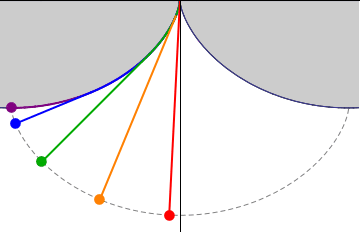$$\require{cancel}$$

# 19.9: The Cycloidal Pendulum

•• Contributed by Jeremy Tatum
• Emeritus Professor (Physics & Astronomy) at University of Victoria

Let us imagine building a wooden construction in the shape of the cycloid$x = a(2 \theta - \sin 2 \theta ) \label{19.9.1}$

$y = 2a \cos ^2 \theta \label{19.9.2}$

shown with the thick line in Figure XIX.10. Now suspend a pendulum of length $$4a$$ from the cusp, and allow it to swing to and fro, partially wrapping itself against the wooden frame as it does so. If the arc length from the cusp to P is $$s$$, then the length of the “free” string is $$4a − s$$, and so the coordinates of the bob at the end of the pendulum are

\begin{align} x &= a(2 \theta - \sin 2 \theta) + (4 a - s) \cos (180 ^\circ - \psi) \nonumber \\ &= a (2 \theta - \sin 2 \theta) + (4a - s) \sin \theta. \nonumber \end{align} \label{19.9.3}

and

\begin{align} y &= 2 a \cos^2 \theta - (4a - s) \sin (180 ^\circ - \psi) \nonumber \\&= 2 a \cos^2 \theta - (4a - s) \cos \theta \nonumber \end{align} \label{19.9.4}

(You will need to remind yourself of the exact meaning of $$\psi$$ and also make use of Equation 19.4.20.) Now Equation 19.4.18 tells us that , and, on substitution of this in equations $$\ref{19.9.3}$$ and $$\ref{19.9.4}$$, we find (after a very little algebra and trigonometry) for the parametric equations to the path described by the bob of the pendulum:

$x = a(2 \theta + \sin 2 \theta \label{19.9.5}$

and

$y = -2 a \cos ^2 \theta. \label{19.9.6}$

Thus the path of the pendulum bob (shown as a dashed line in Figure XIX.10) is a cycloid, and hence its period is independent of its amplitude. (Recall Section 19.5.) Thus the pendulum is isochronous or tautochronous. It is astonishing to learn that Huygens constructed just such a pendulum as long ago as 1673.Five isochronous cycloidal pendula with different amplitudes. All of them are isochronous, meaning they have the same frequency regardless of their amplitudes. Notice the two upper cycloidal arcs which make the bobs describe cycloidal trajectories. Image used with permission (CC BY-SA 4.0; Rem088roy).